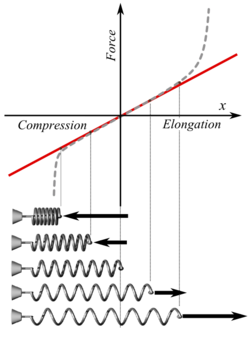## 胡克定律计算器

 我想计算 力(Fx) 弹性系数(k) 形变量(x) 弹簧平衡位置(x0) 弹性系数(k) = N/m 形变量(x) = m 弹簧平衡位置(x0) = m 力(Fx) = NFx = 力

k = 弹性系数

x = 形变量

x0 = 平衡位置